# 漫画：什么是 “哈夫曼树” ？————— 第二天 —————————————————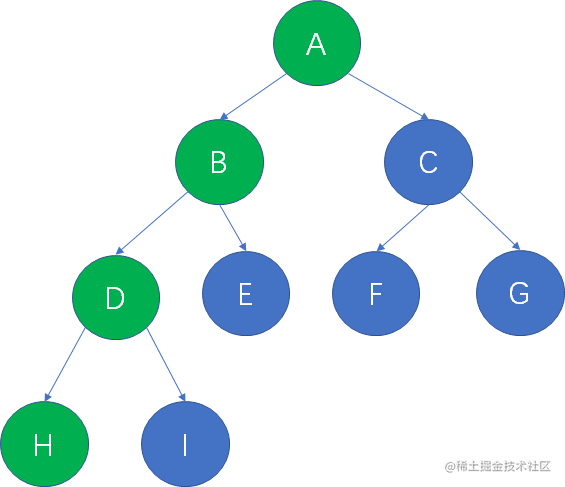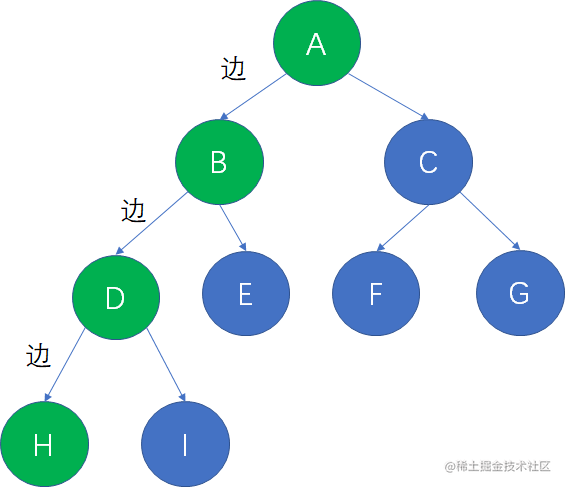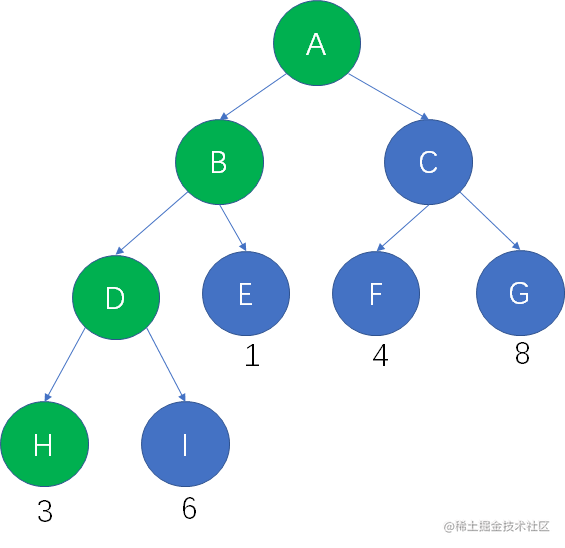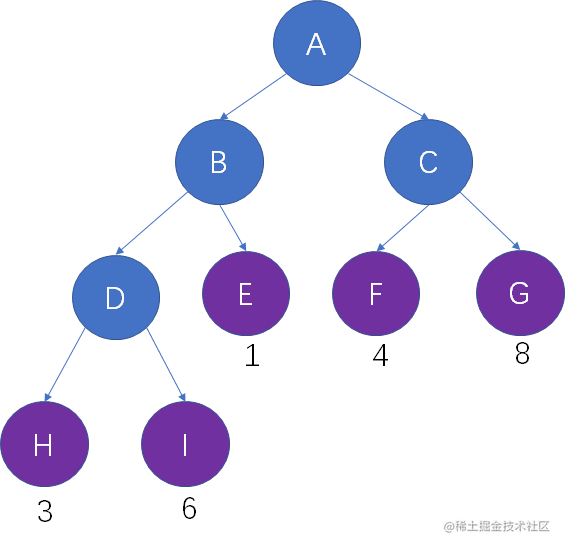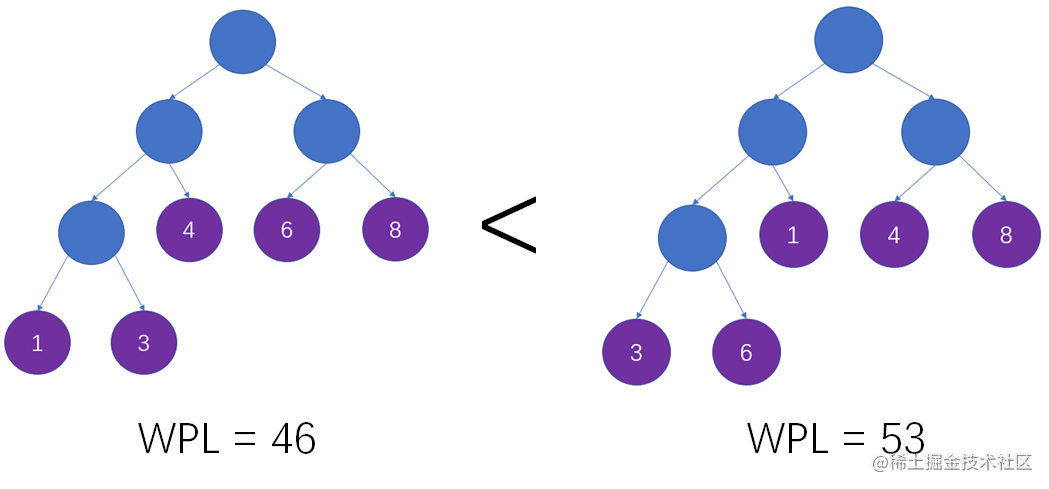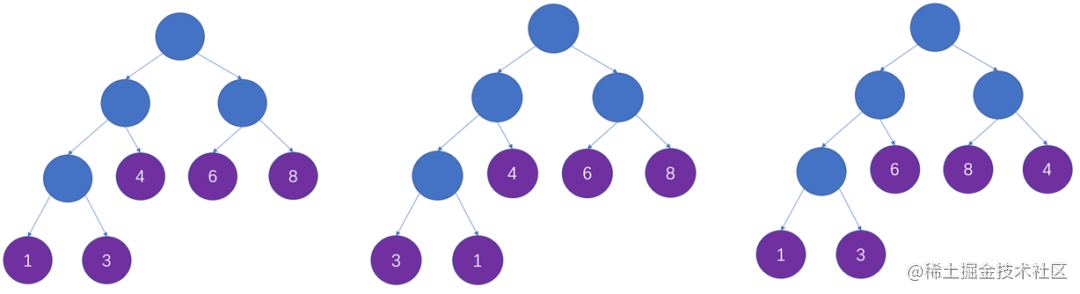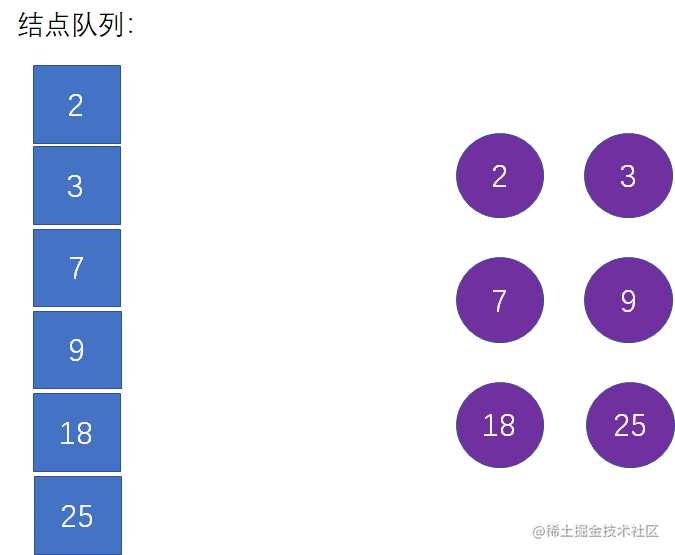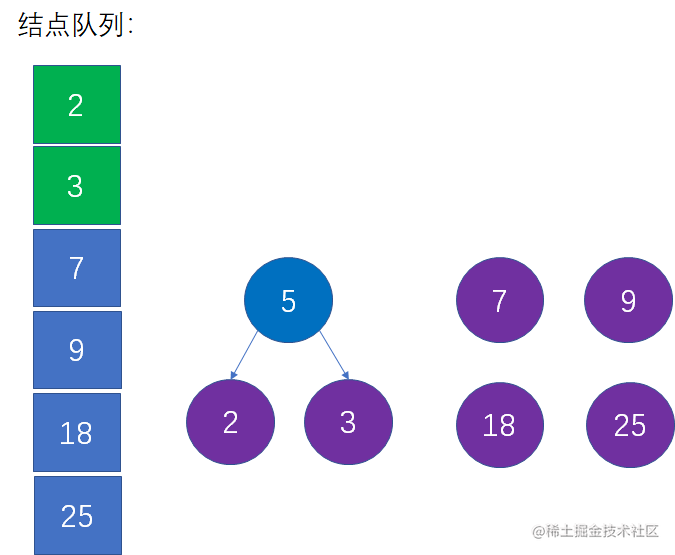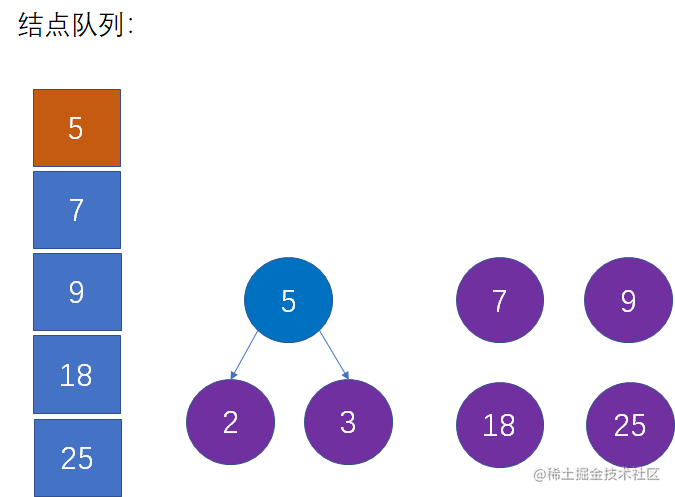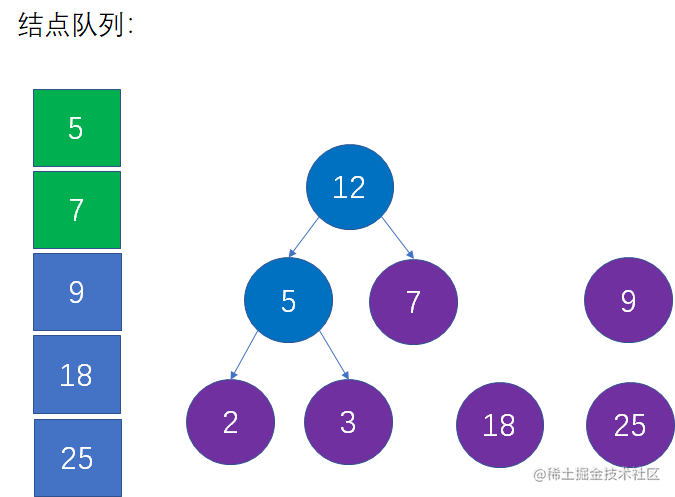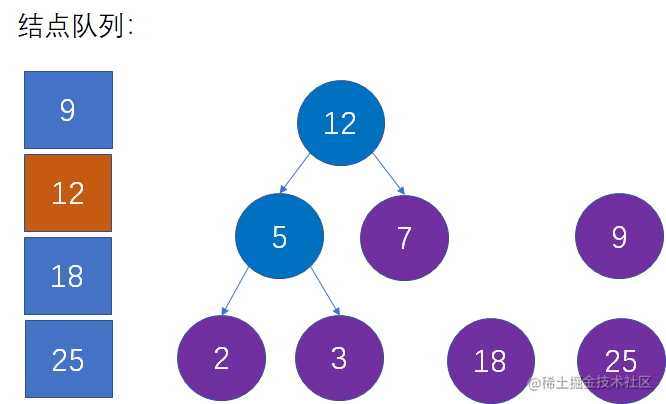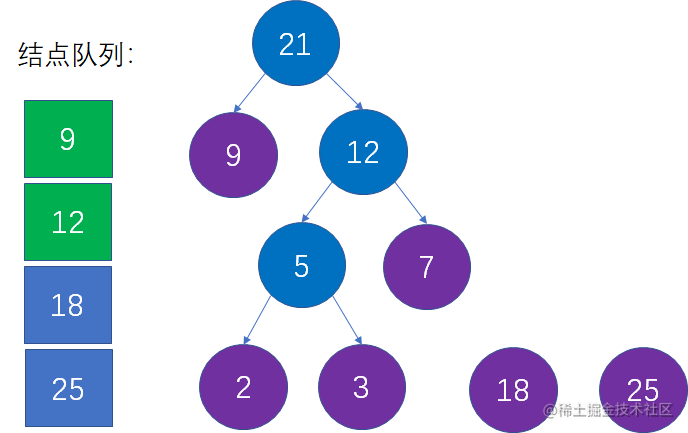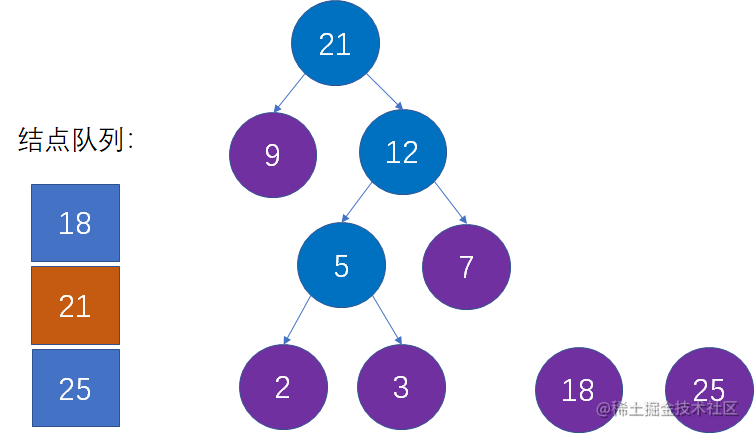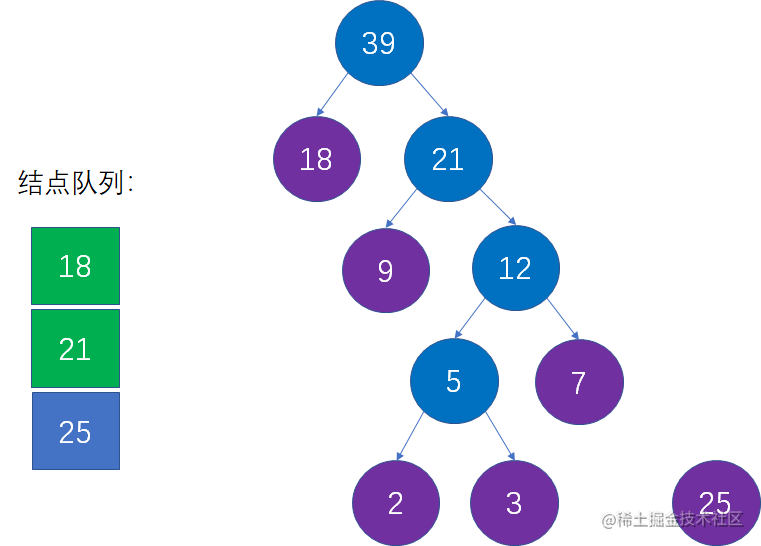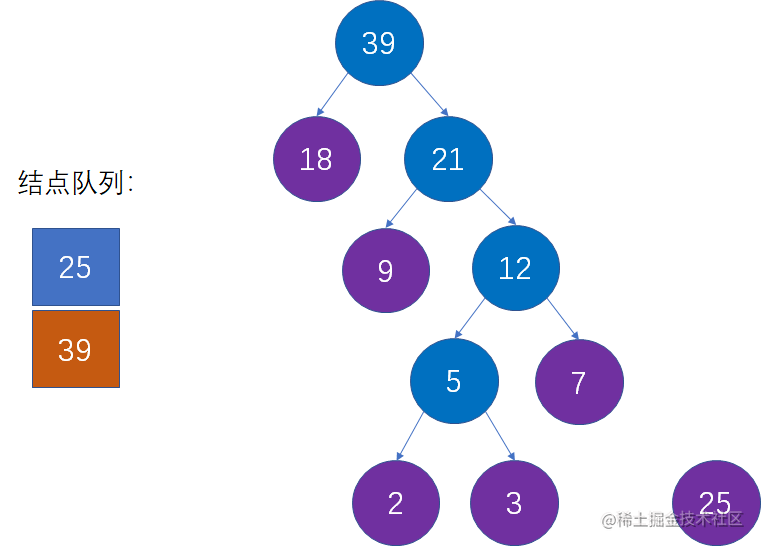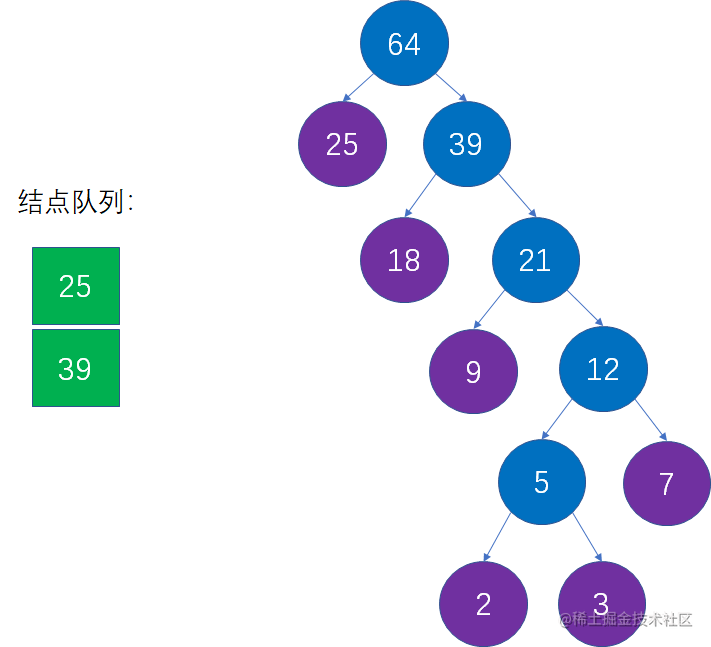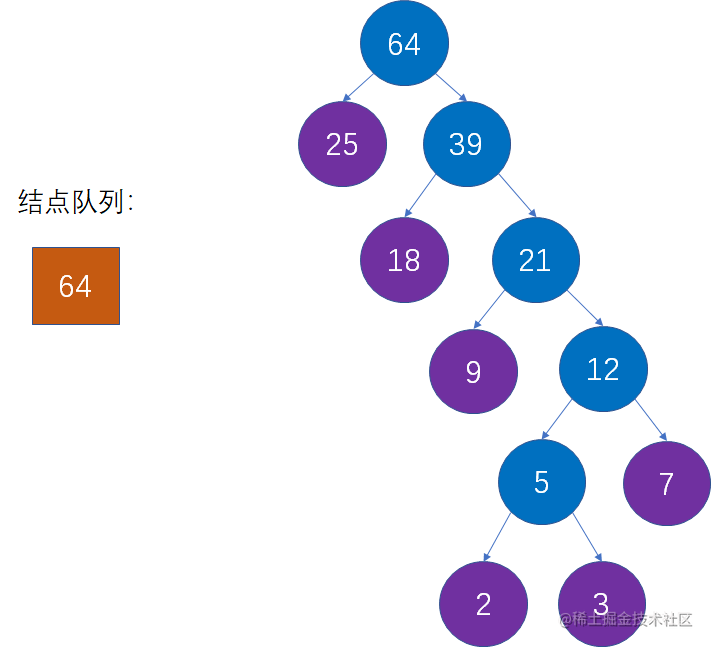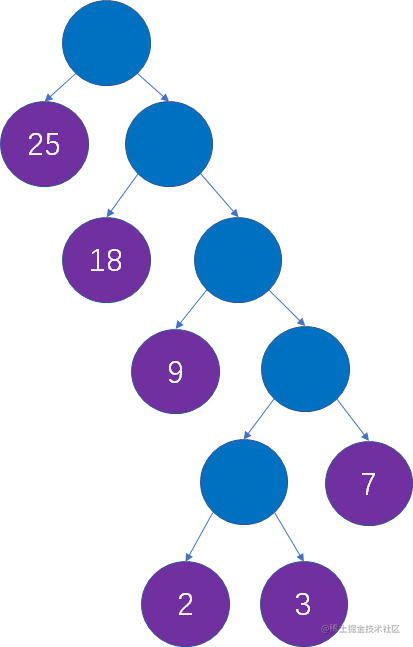``private Node root;private Node[] nodes;//构建哈夫曼树public void createHuffman(int[] weights) {    //优先队列，用于辅助构建哈夫曼树    Queue<Node> nodeQueue = new PriorityQueue<>();    nodes = new Node[weights.length];    //构建森林，初始化nodes数组    for(int i=0; i<weights.length; i++){        nodes[i] = new Node(weights[i]);        nodeQueue.add(nodes[i]);    }    //主循环，当结点队列只剩一个结点时结束    while (nodeQueue.size() > 1) {        //从结点队列选择权值最小的两个结点        Node left = nodeQueue.poll();        Node right = nodeQueue.poll();        //创建新结点作为两结点的父节点        Node parent = new Node(left.weight + right.weight, left, right);        nodeQueue.add(parent);    }    root = nodeQueue.poll();}//按照前序遍历输出public void output(Node head) {    if(head == null){        return;    }    System.out.println(head.weight);    output(head.lChild);    output(head.rChild);}public static class Node implements Comparable<Node>{    int weight;    Node lChild;    Node rChild;    public Node(int weight) {        this.weight = weight;    }    public Node(int weight, Node lChild, Node rChild) {        this.weight = weight;        this.lChild = lChild;        this.rChild = rChild;    }    @Override    public int compareTo(Node o) {        return new Integer(this.weight).compareTo(new Integer(o.weight));    }}public static void main(String[] args) {    int[] weights = {2,3,7,9,18,25};    HuffmanTree huffmanTree = new HuffmanTree();    huffmanTree.createHuffman(weights);    huffmanTree.output(huffmanTree.root);}复制代码``—————END—————``点个[赞]，是对小灰最大的支持！复制代码``